产品中心    Product Center
 明德扬开发板 XILINX官方板 明德扬模块 ALTERA官方板 承接项目 FPGA芯片 培训专题课程 企业培训公司简介 公司荣誉 项目展示
服务网站    Service website
 FPGA论坛 淘宝店铺 FPGA专辑库 FPGA项目承接 B站视频教程
教程中心    Tutorial center
 认识FPGA 案例中心 FPGA开发板介绍 至简原理 问题解答
新闻中心    News Center
 FPGA技术教程 公司公告 招聘信息 培训文章 相关信息# 【案例】 SDRAM读写控制器

1.1 总体设计
1.1.1 概述

SDRAM之所以称为DRAM就是因为他要不断进行刷新才能保留住数据，因为刷新是DRAM最重要的操作。那么要隔多长时间重复一次刷新，目前公认的标准是，存储体中电容的数据有效保存期上限是64ms，也就是每一行刷新的循环周期是64ms
SDRAM是多Bank结构，例如在一个具有两个BankSDRAM模组中，其中一个Bank在进行预充电期间，另一个Bank却马上可以被读取，这样当进行一次读取后，又马上读取已经预充电Bank的数据时，就无需等待而是可以直接读取了，这也就大大提高了存储器的访问速度

1.1.2 设计目标

1.      SDRAM的读写分别由两个按键进行控制，每按下一次，就会产生一个读使能或者写使能；
2.      SDRAM读写模式为全页突发模式，每次写入某个Bank512个数据，在读此Bank的时候，也应该读出相同的512个数据；
3.      SDRAM读写地址都是从地址0开始；
4.     通过一个按键控制读写SDRAM的Bank地址，按键每按下一次，Bank地址加1。

1.1.3 系统结构框图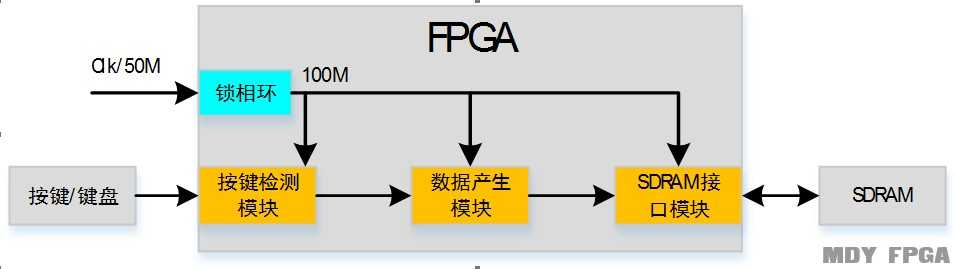1.1.4模块功能

按键检测模块实现功能
1、  将外来异步信号打两拍处理，将异步信号同步化。
2、  实现20ms按键消抖功能。
3、  实现矩阵键盘或者普通案件的检测功能，并输出有效按键信号。

1、产生工程所需要的100M时钟。

数据产生模块实现功能
1、  通过按键控制产生读/写请求。
2、  通过按键控制Bank地址选择。
3、  产生地址和写数据。

SDRAM接口模块实现功能
1、  接收上游模块发送的读/写请求、Bank地址、行地址和写数据，产生SDRAM的控制时序。

1.1.5顶层信号
 信号名 I/O 位宽 定义 clk I 1 系统工作时钟 50M rst_n I 1 系统复位信号，低电平有效 Key I 4 4位按键信号，开发板按键为矩阵键盘时，不需要该信号 Key_col I 4 4位矩阵键盘列信号，默认高电平，开发板按键为普通按键时，不需要该信号 Key_row O 4 4位矩阵键盘行信号，默认低电平，开发板按键为普通按键时，不需要该信号 dq I/O 16 SDRAM数据总线，既能作为数据输出，也能作为数据输入。 cke O 1 SDRAM时钟使能信号，决定是否启用clk输入，为高电平时，时钟有效。 cs O 1 SDRAM片选信号，决定设备内是否启用命令输入，当cs为低时启用，当cs为高时禁用命令输入。 ras O 1 行地址选通信号，低电平有效 cas O 1 列地址选通信号，低电平有效 we O 1 写使能信号，低电平有效 dqm O 2 数据掩码，控制I/O的高低字节，低电平有效。例如：2’b10，表示数据高字节无效，低字节有效。 sd_addr O 13 SDRAM地址信号。 sd_bank O 2 Bank地址选择信号，通过该信号决定哪个Bank正处于激活、读、写、预充电等命令期间。 sd_clk O 1 SDRAM输入时钟，除cke外，SDRAM的所有输入与该引脚的上升沿同步获得。

1.1.6三态门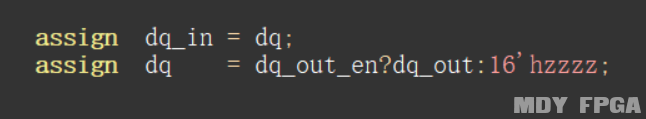1.1.7参考代码

1. module sdram_top(
2.     clk      ,
3.     rst_n    ,
4.     key      ,
5.     dq       ,
6.     cke      ,
7.     cs       ,
8.     ras      ,
9.     cas      ,
10.     we       ,
11.     dqm      ,
13.     sd_bank  ,
14.     sd_clk
15.     );

16.     input              clk     ;
17.     input              rst_n   ;
18.     input  [3:0]       key     ;
19.     inout  [15:0]      dq      ;
20.     output             cke     ;
21.     output             cs      ;
22.     output             ras     ;
23.     output             cas     ;
24.     output             we      ;
25.     output [1 :0]      dqm     ;
27.     output [1 :0]      sd_bank ;
28.     output             sd_clk  ;

29.     wire               cke     ;
30.     wire               cs      ;
31.     wire               ras     ;
32.     wire               cas     ;
33.     wire               we      ;
34.     wire  [1 :0]       dqm     ;
36.     wire  [1 :0]       sd_bank ;
37.     wire               sd_clk  ;

38.     wire              clk_100m ;
39.     wire              wr_ack   ;
40.     wire              rd_ack   ;
41.     wire              wr_req   ;
42.     wire              rd_req   ;
43.     wire [1 :0]       bank     ;
45.     wire [15:0]       wdata    ;
46.     wire [15:0]       rdata    ;
47.     wire              rdata_vld;
48.     wire [3:0 ]       key_vld  ;

49.     wire [15:0]       dq_in    ;
50.     wire [15:0]       dq_out   ;
51.     wire              dq_out_en;

52.     assign  dq_in = dq;
53.     assign  dq    = dq_out_en?dq_out:16'hzzzz;
54.

55.     pll_100m uut_pll(
56.             .inclk0    (clk      ),
57.             .c0        (clk_100m )
58.     );

59.     key_module uut_key(
60.         .clk       (clk_100m ),
61.         .rst_n     (rst_n    ),
62.         .key_in    (key      ),
63.         .key_vld   (key_vld  ),
64.     );
65.
66.     data_ctrl uut_ctrl(
67.         .clk       (clk_100m ),
68.         .rst_n     (rst_n    ),
69.         .key_vld   (key_vld  ),
70.         .wr_ack    (wr_ack   ),
71.         .rd_ack    (rd_ack   ),
72.         .wr_req    (wr_req   ),
73.         .rd_req    (rd_req   ),
74.         .bank      (bank     ),
76.         .wdata     (wdata    )
77.         );
78.
79.     sdram_intf uut_sdram(
80.         .clk       (clk_100m ),
81.         .rst_n     (rst_n    ),
82.         .wr_req    (wr_req   ),
83.         .rd_req    (rd_req   ),
84.         .bank      (bank     ),
86.         .wdata     (wdata    ),
87.         .dq_in     (dq_in    ),
88.         .dq_out    (dq_out   ),
89.         .dq_out_en (dq_out_en),
90.         .wr_ack    (wr_ack   ),
91.         .rd_ack    (rd_ack   ),
92.         .rdata     (rdata    ),
93.         .rdata_vld (rdata_vld),
94.         .cke       (cke      ),
95.         .cs        (cs       ),
96.         .ras       (ras      ),
97.         .cas       (cas      ),
98.         .we        (we       ),
99.         .dqm       (dqm      ),
101.         .sd_bank   (sd_bank  ),
102.         .sd_clk    (sd_clk   )
103.
104.     );

105.     endmodule

1.2 按键检测模块设计
1.2.1接口信号

 信号 接口方向 定义 clk 输入 系统时钟 rst_n 输入 低电平复位信号 key_col 输入 矩阵键盘列输入信号 Key_row 输出 矩阵键盘行输出信号 Key_en 输出 按键按下位置指示信号

 信号 接口方向 定义 clk 输入 系统时钟 rst_n 输入 低电平复位信号 Key_in 输入 按键输入信号 Key_vld 输出 按键按下指示信号

1.2.2 设计思路

1.2.3 参考代码
1.    //矩阵键盘
1. always  @(posedge clk or negedge rst_n)begin
2.     if(rst_n==1'b0)begin
3.         key_col_ff0 <= 4'b1111;
4.         key_col_ff1 <= 4'b1111;
5.     end
6.     else begin
7.         key_col_ff0 <= key_col    ;
8.         key_col_ff1 <= key_col_ff0;
9.     end
10. end

11. always @(posedge clk or negedge rst_n) begin
12.     if (rst_n==0) begin
13.         shake_cnt <= 0;
14.     end
16.         if(end_shake_cnt)
17.             shake_cnt <= 0;
18.         else
19.             shake_cnt <= shake_cnt+1 ;
20.    end
21. end
23. assign end_shake_cnt = add_shake_cnt  && shake_cnt == TIME_20MS-1 ;

24. always  @(posedge clk or negedge rst_n)begin
25.     if(rst_n==1'b0)begin
26.         state_c <= CHK_COL;
27.     end
28.     else begin
29.         state_c <= state_n;
30.     end
31. end

32. always  @(*)begin
33.     case(state_c)
34.         CHK_COL: begin
35.                      if(col2row_start )begin
36.                          state_n = CHK_ROW;
37.                      end
38.                      else begin
39.                          state_n = CHK_COL;
40.                      end
41.                  end
42.         CHK_ROW: begin
43.                      if(row2del_start)begin
44.                          state_n = DELAY;
45.                      end
46.                      else begin
47.                          state_n = CHK_ROW;
48.                      end
49.                  end
50.         DELAY :  begin
51.                      if(del2wait_start)begin
52.                          state_n = WAIT_END;
53.                      end
54.                      else begin
55.                          state_n = DELAY;
56.                      end
57.                  end
58.         WAIT_END: begin
59.                      if(wait2col_start)begin
60.                          state_n = CHK_COL;
61.                      end
62.                      else begin
63.                          state_n = WAIT_END;
64.                      end
65.                   end
66.        default: state_n = CHK_COL;
67.     endcase
68. end
69. assign col2row_start = state_c==CHK_COL  && end_shake_cnt;
70. assign row2del_start = state_c==CHK_ROW  && row_index==3 && end_row_cnt;
71. assign del2wait_start= state_c==DELAY    && end_row_cnt;
72. assign wait2col_start= state_c==WAIT_END && key_col_ff1==4'hf;

73. always  @(posedge clk or negedge rst_n)begin
74.     if(rst_n==1'b0)begin
75.         key_row <= 4'b0;
76.     end
77.     else if(state_c==CHK_ROW)begin
78.         key_row <= ~(1'b1 << row_index);
79.     end
80.     else begin
81.         key_row <= 4'b0;
82.     end
83. end

84. 普通键盘

85. always @(posedge clk or negedge rst_n) begin
86.     if (rst_n==0) begin
87.         row_index <= 0;
88.     end
90.         if(end_row_index)
91.             row_index <= 0;
92.         else
93.             row_index <= row_index+1 ;
94.    end
95.    else if(state_c!=CHK_ROW)begin
96.        row_index <= 0;
97.    end
98. end
99. assign add_row_index = state_c==CHK_ROW && end_row_cnt;
100. assign end_row_index = add_row_index  && row_index == 4-1 ;

101. always @(posedge clk or negedge rst_n) begin
102.     if (rst_n==0) begin
103.         row_cnt <= 0;
104.     end
106.         if(end_row_cnt)
107.             row_cnt <= 0;
108.         else
109.             row_cnt <= row_cnt+1 ;
110.    end
111. end
112. assign add_row_cnt = state_c==CHK_ROW || state_c==DELAY;
113. assign end_row_cnt = add_row_cnt  && row_cnt == 16-1 ;

114. always  @(posedge clk or negedge rst_n)begin
115.     if(rst_n==1'b0)begin
116.         key_col_get <= 0;
117.     end
118.     else if(state_c==CHK_COL && end_shake_cnt ) begin
119.         if(key_col_ff1==4'b1110)
120.             key_col_get <= 0;
121.         else if(key_col_ff1==4'b1101)
122.             key_col_get <= 1;
123.         else if(key_col_ff1==4'b1011)
124.             key_col_get <= 2;
125.         else
126.             key_col_get <= 3;
127.     end
128. end

129. always  @(posedge clk or negedge rst_n)begin
130.     if(rst_n==1'b0)begin
131.         key_out <= 0;
132.     end
133.     else if(state_c==CHK_ROW && end_row_cnt)begin
134.         key_out <= {row_index,key_col_get};
135.     end
136.     else begin
137.         key_out <= 0;
138.     end
139. end

140. always  @(posedge clk or negedge rst_n)begin
141.     if(rst_n==1'b0)begin
142.         key_vld <= 1'b0;
143.     end
144.     else if(state_c==CHK_ROW && end_row_cnt && key_col_ff1[key_col_get]==1'b0)begin
145.         key_vld <= 1'b1;
146.     end
147.     else begin
148.         key_vld <= 1'b0;
149.     end
150. end

151. always  @(*)begin
152.     if(rst_n==1'b0)begin
153.         key_en = 0;
154.     end
155.     else if(key_vld && key_out==0)begin
156.         key_en = 4'b0001;
157.     end
158.     else if(key_vld && key_out==1)begin
159.         key_en = 4'b0010;
160.     end
161.     else if(key_vld && key_out==2)begin
162.         key_en = 4'b0100;
163.     end
164.     else begin
165.         key_en = 0;
166.     end
167. end

168. endmodule

1.3 锁相环
1.3.1 接口信号
 信号名 I/O 位宽 定义 Inclk0 I 1 输入时钟50M C0 O 1 输出时钟100M

1.3.2 设计思路

1.4 数据产生模块设计
1.4.1接口信号
 信号名 I/O 位宽 定义 clk I 1 工作时钟 100M rst_n I 1 系统复位信号，低电平有效 Key_vld I 4 按键有效指示信号 Wr_ack I 1 写数据响应 rd_ack I 1 读数据响应 Wr_req O 1 写数据请求 rd_req O 1 读数据响应 bank O 2 Bank地址选择信号 addr O 13 SDRAM地址信号 Wdata O 16 SDRAM写数据

1.4.2设计思路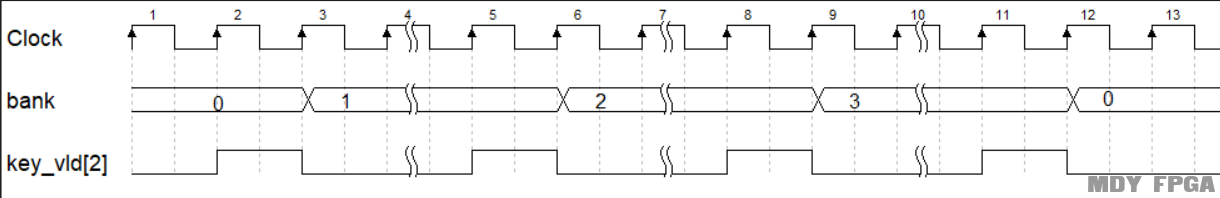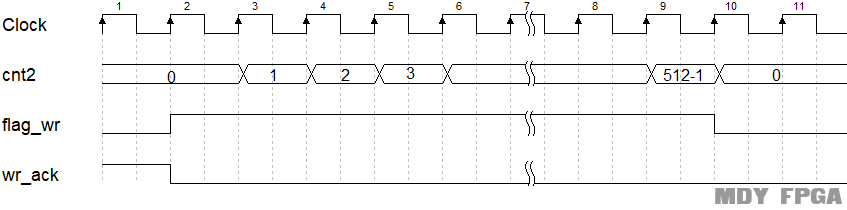1.4.3参考代码
1. always  @(posedge clk or negedge rst_n)begin
2.     if(rst_n==1'b0)begin
3.         wr_req <= 0;
4.     end
5.     else if(key_vld==1)begin
6.         wr_req <= 1;
7.     end
8.     else if(wr_ack)begin
9.         wr_req <= 0;
10.     end
11. end

12. always  @(posedge clk or negedge rst_n)begin
13.     if(rst_n==1'b0)begin
14.         rd_req <= 0;
15.     end
16.     else if(key_vld==1)begin
17.         rd_req <= 1;
18.     end
19.     else if(rd_ack)begin
20.         rd_req <= 0;
21.     end
22. end

23. always @(posedge clk or negedge rst_n) begin
24.     if (rst_n==0) begin
25.         bank <= 0;
26.     end
28.         if(end_bank)
29.             bank <= 0;
30.         else
31.             bank <= bank+1 ;
32.    end
33. end
35. assign end_bank = add_bank  && bank == 4-1 ;

36. always  @(*)begin
37.     if(flag_wr)begin
38.         wdata = {7'b0,cnt2};
39.     end
40.     else begin
41.         wdata = 0;
42.     end
43. end

44. always  @(posedge clk or negedge rst_n)begin
45.     if(rst_n==1'b0)begin
46.         flag_wr <= 0;
47.     end
48.     else if(wr_ack)begin
49.         flag_wr <= 1;
50.     end
51.     else if(end_cnt2)begin
52.         flag_wr <= 0;
53.     end
54. end

55. always @(posedge clk or negedge rst_n) begin
56.     if (rst_n==0) begin
57.         cnt2 <= 0;
58.     end
60.         if(end_cnt2)
61.             cnt2 <= 0;
62.         else
63.             cnt2 <= cnt2+1 ;
64.    end
65. end
67. assign end_cnt2 = add_cnt2  && cnt2 == 512-1 ;

69. endmodule

1.5 SDRAM接口模块设计
1.5.1接口信号
 信号名 I/O 位宽 定义 clk I 1 1工作时钟  100M rst_n I 1 系统复位信号，低电平有效 Wr_req I 1 写请求 rd_req I 1 读请求 bank I 2 输入bank地址 addr I 13 地址信号 Wdata I 16 写数据 dq_in I 16 SDRAM数据输入 dq_out O 16 写SDRAM数据信号 dq_out_en O 1 三态门使能 Wr_ack O 1 写响应 rd_ack O 1 读响应 rdata O 16 读数据 rdata_vld O 1 读数据有效指示信号 Cke O 1 SDRAM时钟使能信号，决定是否启用clk输入，为高电平时，时钟有效。 Cs O 1 SDRAM片选信号，决定设备内是否启用命令输入，当cs为低时启用，当cs为高时禁用命令输入。 ras O 1 行地址选通信号，低电平有效 Cas O 1 列地址选通信号，低电平有效 We O 1 写使能信号，低电平有效 dqm O 2 数据掩码，控制I/O的高低字节，低电平有效。例如：2’b10，表示数据高字节无效，低字节有效。 Sd_addr O 13 SDRAM地址信号。 Sd_bank O 2 Bank地址选择信号，通过该信号决定哪个Bank正处于激活、读、写、预充电等命令期间。 Sd_clk O 1 SDRAM输入时钟，除cke外，SDRAM的所有输入与该引脚的上升沿同步获得。

1.5.2SDRAM工作流程

SDRAM初始化
SDRAM内部有一个逻辑控制单元，并且有一个模式寄存器为其提供控制参数。每次开机时SDRAM都要先对这个控制逻辑核心进行初始化。SDRAM必须以预定义的方式启动和初始化。在电源同时作用于VddVddq后开始初始化SDRAM，此时的时钟稳定并且将数据掩码和时钟使能信号拉高。在向SDRAM发送命令之前需要有100us的延时，此时SDRAM不执行任何操作。在100us延时满足后，需要对Bank进行预充电，在此期间所有的Bank处于空闲状态。预充电之后会有至少两个自刷新操作，完成自刷新便可以对SDRAM进行模式寄存器配置。下面是初始化的时序图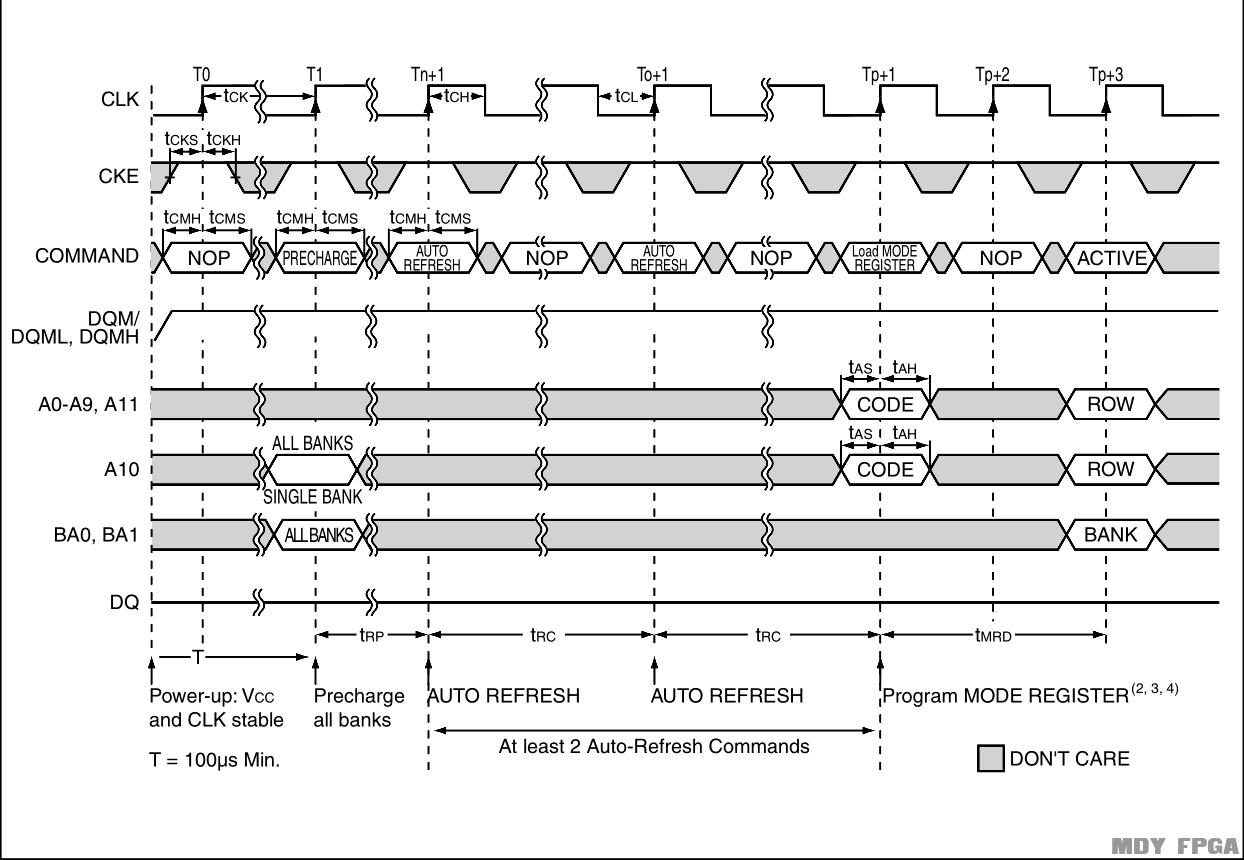SDRAM行激活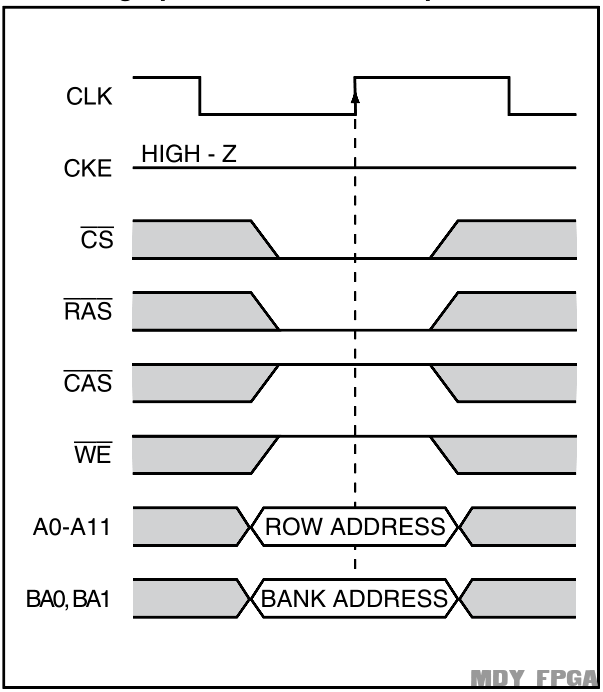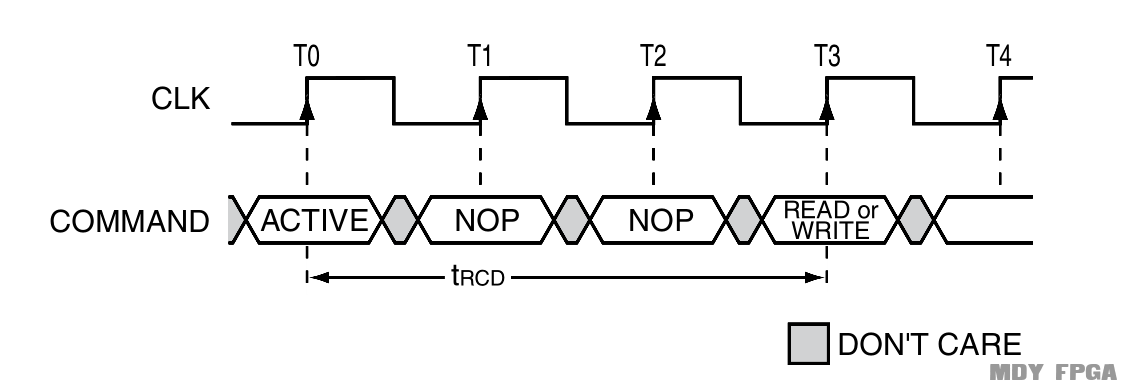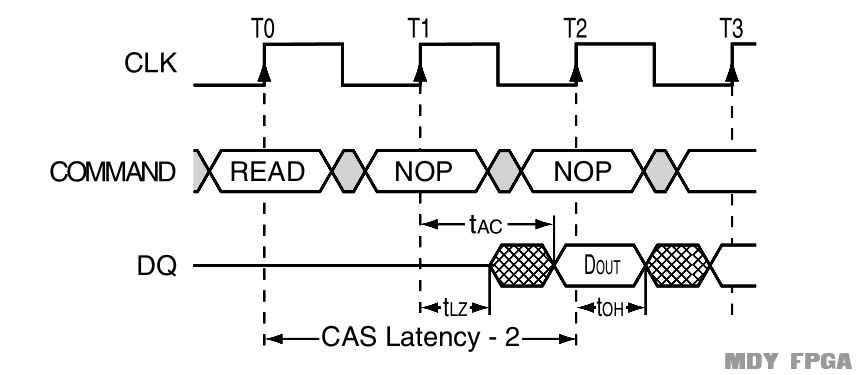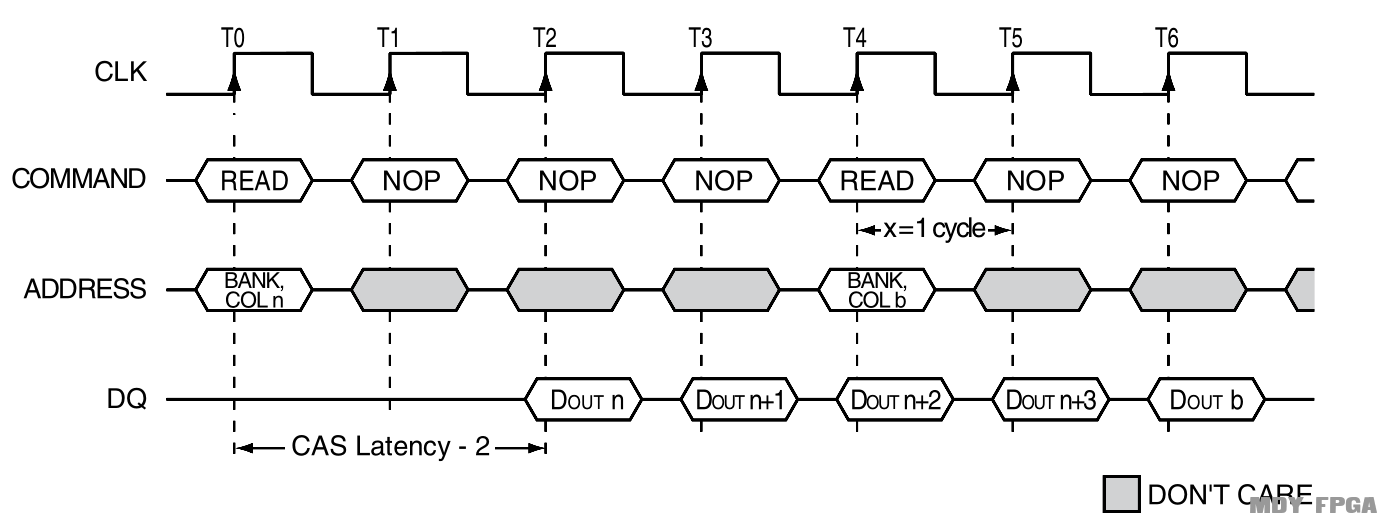1.5.3设计思路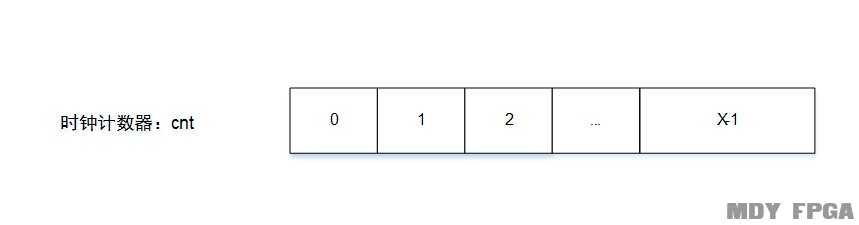当前状态 计数器数多少个 空操作（NOP） 20000 预充电（PER） 2 自刷新（REF） 7 加载模式寄存器（MOD） 2 激活（ACT） 2 读/写数据（RED/WIR） 512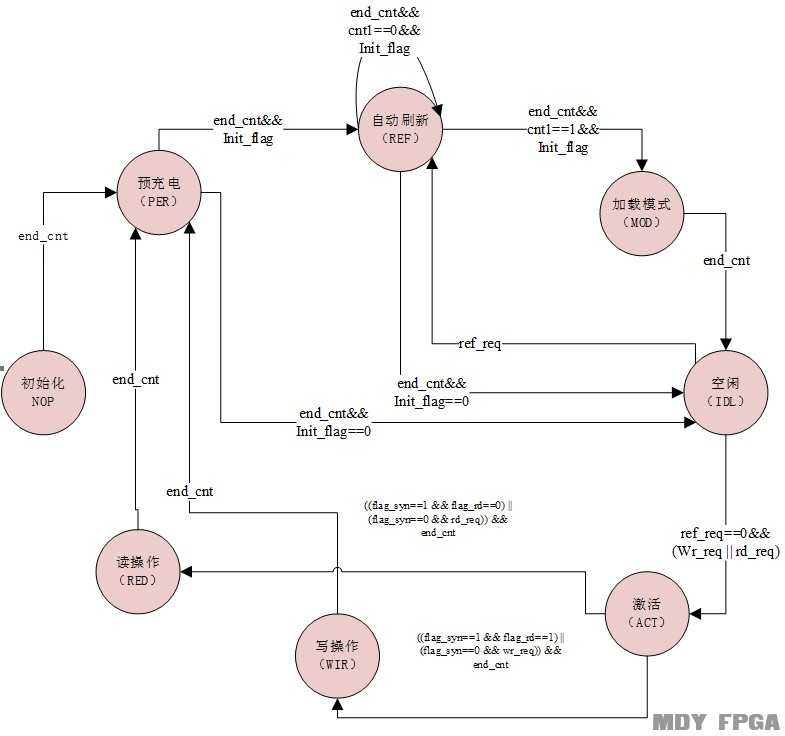1、  延时100us之后，进入到预充电状态。

1、  如果处于初始化阶段，两个时钟周期之后，跳转到自刷新状态。
2、  如果不是初始化阶段，两个时钟周期之后，跳转到空闲状态。

1、  如果处于初始化状态，7个时钟周期之后，跳转到自刷新状态。
2、  如果处于初始化状态，并且已经进行过一次初始化，7个时钟周期之后，跳转到加载模式寄存器状态。
3、  如果不是初始化阶段，7个时钟周期之后，跳转到空闲状态。

1、  2个时钟周期之后，进入到空闲状态。

1、  如果收到自刷新请求，则跳转到自刷新状态。
2、  如果自刷新请求无效，收到读/写请求，则跳转到激活状态。

1、  当读写请求不同时的时候，接收到读请求，则跳转到读状态。
2、  当读写请求不同时的时候，接收到写请求，则跳转到写状态
3、  当读写请求同时到达的时候，第一次来的时候，首先响应读请求，跳转到读状态
4、  当读写请求同时到达，但不是第一次同时有效的时候，则根据上一次执行的操作进行判断，如果上一次执行的读操作，则这次执行写操作，跳转到写状态；如果上一次执行的写操作，则这次执行读操作，跳转到读状态。

1、  写数据完成，就进入到预充电状态。

1、  读数据完成，就进入到预充电状态。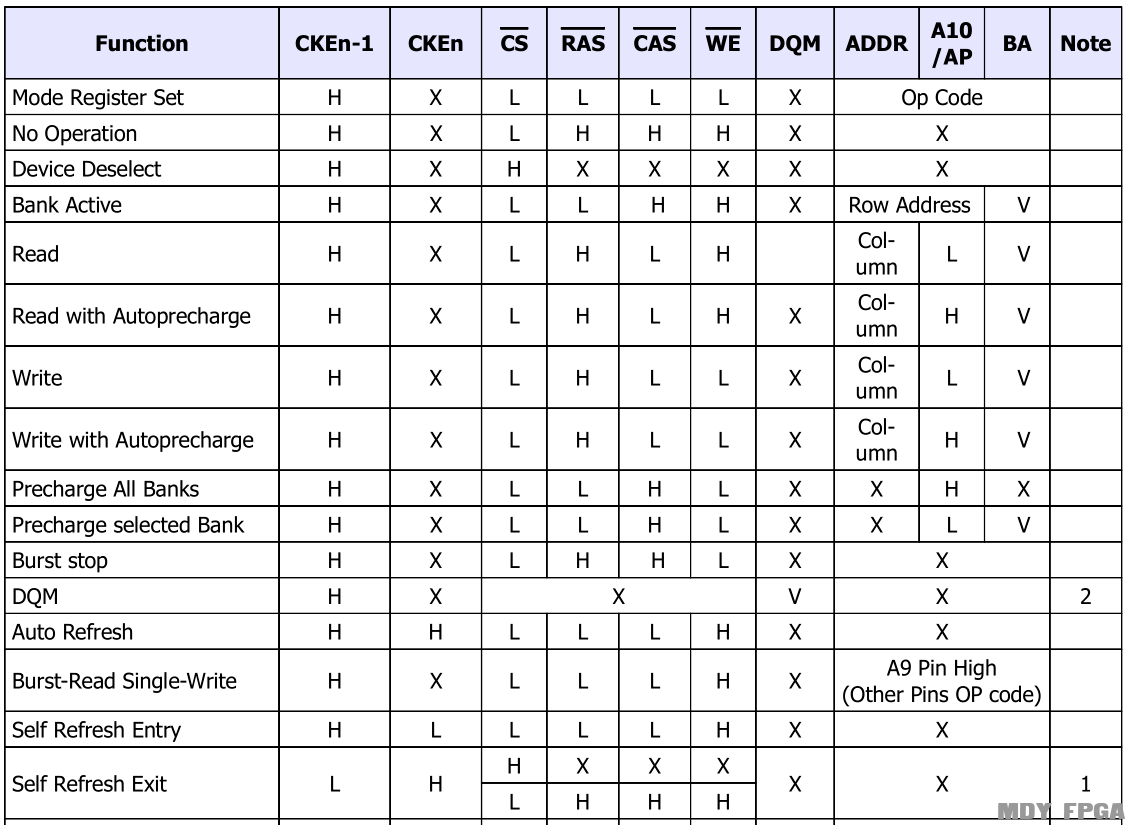Bank选择信号sd_bank：初始状态为2’b00，表示选择Bank0；在激活阶段、读阶段和写阶段，该信号由输入得bank信号决定。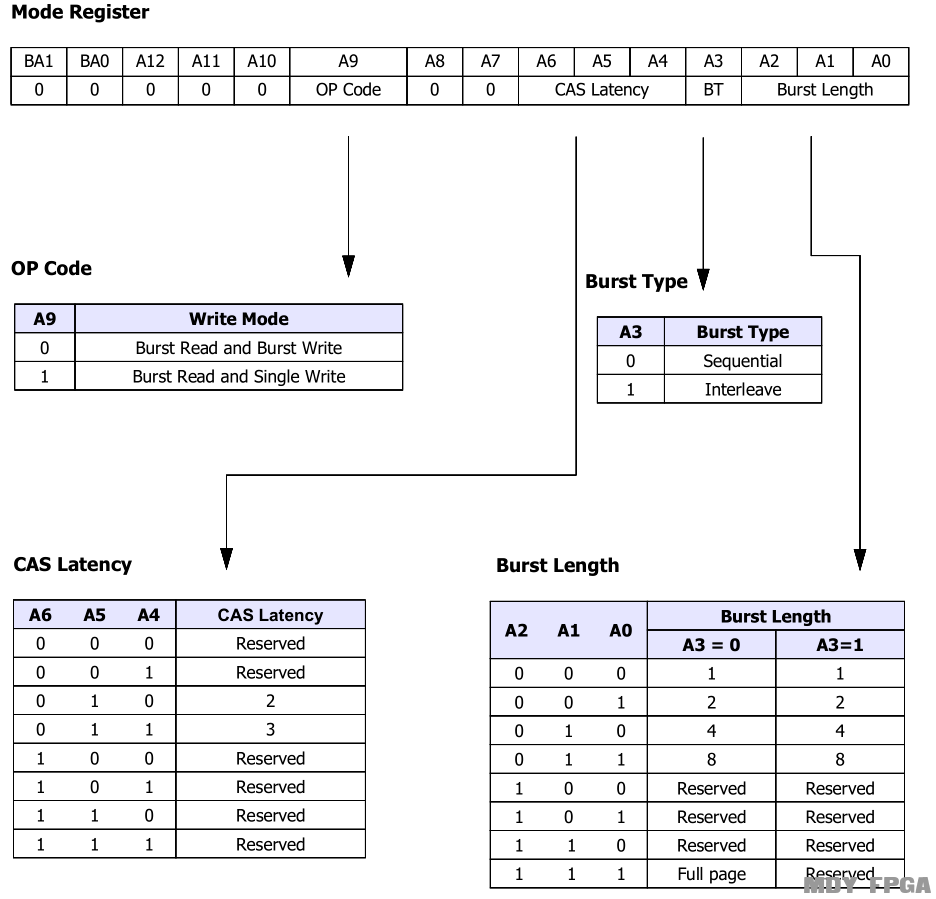1.5.4参考代码
1.     parameter NOP       = 4'b0000 ;
2.     parameter PER       = 4'b0001 ;
3.     parameter REF       = 4'b0010 ;
4.     parameter MOD       = 4'b0100 ;
5.     parameter IDL       = 4'b1000 ;
6.     parameter ACT       = 4'b0011 ;
7.     parameter RED       = 4'b0110 ;
8.     parameter WIR       = 4'b1100 ;
9.
10.     parameter NOP_CMD   = 4'b0111 ;
11.     parameter PER_CMD   = 4'b0010 ;
12.     parameter REF_CMD   = 4'b0001 ;
13.     parameter MOD_CMD   = 4'b0000 ;
14.     parameter ACT_CMD   = 4'b0011 ;
15.     parameter RED_CMD   = 4'b0101 ;
16.     parameter WIR_CMD   = 4'b0100 ;
17.
18.     parameter ALL_BANK  = 13'b001_0_00_000_0_000;
19.     parameter CODE      = 13'b000_0_00_010_0_111;
20.
21.     parameter TIME_780  = 780     ;
22.     parameter TIME_WAIT = 10000   ;
23.     parameter TIME_TRP  = 2       ;
24.     parameter TIME_TRC  = 7       ;
25.     parameter TIME_TMRD = 2       ;
26.     parameter TIME_TRCD = 2       ;
27.     parameter TIME_512  = 512     ;

28.     input            clk          ;
29.     input            rst_n        ;
30.     input            wr_req       ;
31.     input            rd_req       ;
32.     input  [1 :0]    bank         ;
34.     input  [15:0]    wdata        ;
35.     input  [15:0]    dq_in        ;

36.     output [15:0]    dq_out       ;
37.     output           dq_out_en    ;
38.     output           wr_ack       ;
39.     output           rd_ack       ;
40.     output [15:0]    rdata        ;
41.     output           rdata_vld    ;
42.     output           cke          ;
43.     output           cs           ;
44.     output           ras          ;
45.     output           cas          ;
46.     output           we           ;
47.     output [1 :0]    dqm          ;
49.     output [1 :0]    sd_bank      ;
50.     output           sd_clk       ;

51.     reg    [15:0]    dq_out       ;
52.     reg              dq_out_en    ;
53.     reg              flag_syn     ;
54.     wire             wr_ack       ;
55.     wire             rd_ack       ;
56.     reg    [15:0]    rdata        ;
57.     reg              rdata_vld    ;
58.     reg              cke          ;
59.     wire             cs           ;
60.     wire             ras          ;
61.     wire             cas          ;
62.     wire             we           ;
63.     reg    [1 :0]    dqm          ;
65.     reg    [1 :0]    sd_bank      ;
66.     wire             sd_clk       ;

67.     reg    [3:0]     state_c      ;
68.     reg    [3:0]     state_n      ;
69.     wire             nop2per_start;
70.     wire             per2ref_start;
71.     wire             per2idl_start;
72.     wire             ref2ref_start;
73.     wire             ref2mod_start;
74.     wire             ref2idl_start;
75.     wire             mod2idl_start;
76.     wire             idl2ref_start;
77.     wire             idl2act_start;
78.     wire             act2red_start;
79.     wire             act2wir_start;
80.     wire             red2per_start;
81.     wire             wir2per_start;
82.     reg    [3:0]     conmand      ;
83.     reg    [13:0]    cnt          ;
85.     wire             end_cnt      ;
86.     reg    [1:0]     cnt1         ;
88.     wire             end_cnt1     ;
89.     reg    [9:0]     cnt_780      ;
91.     wire             end_cnt_780  ;
92.     reg    [13:0]    x            ;
93.     reg              init_flag    ;
94.     reg              ref_req      ;
95.     wire             ref_ack      ;
96.     reg              flag_rd      ;
97.     wire             rd_en        ;
98.     reg              rdata_vld_ff0;
99.     reg              rdata_vld_ff1;
100.     reg              rdata_vld_ff2;

101. always  @(posedge clk or negedge rst_n)begin
102.     if(rst_n==1'b0)begin
103.         state_c <= NOP;
104.     end
105.     else begin
106.         state_c <= state_n;
107.     end
108. end

109. always @(*)begin
110.     case(state_c)
111.         NOP:begin
112.             if(nop2per_start)begin
113.                 state_n = PER;
114.             end
115.             else begin
116.                 state_n = state_c;
117.             end
118.         end
119.         PER:begin
120.             if(per2ref_start)begin
121.                 state_n = REF;
122.             end
123.             else if(per2idl_start)begin
124.                 state_n = IDL;
125.             end
126.             else begin
127.                 state_n = state_c;
128.             end
129.         end
130.         REF:begin
131.             if(ref2ref_start)begin
132.                 state_n = REF;
133.             end
134.             else if(ref2mod_start)begin
135.                 state_n = MOD;
136.             end
137.             else if(ref2idl_start)begin
138.                 state_n = IDL;
139.             end
140.             else begin
141.                 state_n = state_c;
142.             end
143.         end
144.         MOD:begin
145.             if(mod2idl_start)begin
146.                 state_n = IDL;
147.             end
148.             else begin
149.                 state_n = state_c;
150.             end
151.         end
152.         IDL:begin
153.             if(idl2ref_start)begin
154.                 state_n = REF;
155.             end
156.             else if(idl2act_start)begin
157.                 state_n = ACT;
158.             end
159.             else begin
160.                 state_n = state_c;
161.             end
162.         end
163.         ACT:begin
164.             if(act2red_start)begin
165.                 state_n = RED;
166.             end
167.             else if(act2wir_start)begin
168.                 state_n = WIR;
169.             end
170.             else begin
171.                 state_n = state_c;
172.             end
173.         end
174.         RED:begin
175.             if(red2per_start)begin
176.                 state_n = PER;
177.             end
178.             else begin
179.                 state_n = state_c;
180.             end
181.         end
182.         WIR:begin
183.             if(wir2per_start)begin
184.                 state_n = PER;
185.             end
186.             else begin
187.                 state_n = state_c;
188.             end
189.         end
190.         default:begin
191.             state_n = IDL;
192.         end
193.     endcase
194. end

195. assign nop2per_start = state_c==NOP && end_cnt;
196. assign per2ref_start = state_c==PER && init_flag==1 && end_cnt;
197. assign per2idl_start = state_c==PER && init_flag==0 && end_cnt;
198. assign ref2ref_start = state_c==REF && init_flag==1 && cnt1==0 && end_cnt;
199. assign ref2mod_start = state_c==REF && init_flag==1 && end_cnt1;
200. assign ref2idl_start = state_c==REF && init_flag==0 && end_cnt;
201. assign mod2idl_start = state_c==MOD && end_cnt;
202. assign idl2ref_start = state_c==IDL && ref_req;
203. assign idl2act_start = state_c==IDL && ref_req==0 && (wr_req || rd_req);
204. assign act2red_start = state_c==ACT && ((flag_syn==1 && flag_rd==0) || (flag_syn==0 && rd_req)) && end_cnt;
205. assign act2wir_start = state_c==ACT && ((flag_syn==1 && flag_rd==1) || (flag_syn==0 && wr_req)) && end_cnt;
206. assign red2per_start = state_c==RED && end_cnt;
207. assign wir2per_start = state_c==WIR && end_cnt;

208. always @(posedge clk or negedge rst_n)begin
209.     if(!rst_n)begin
210.         cnt <= 0;
211.     end
213.         if(end_cnt)
214.             cnt <= 0;
215.         else
216.             cnt <= cnt + 1;
217.     end
218. end

220. assign end_cnt = add_cnt && cnt== x-1;

221. always  @(posedge clk or negedge rst_n)begin
222.     if(rst_n==1'b0)begin
223.         init_flag <= 1;
224.     end
225.     else if(mod2idl_start)begin
226.         init_flag <= 0;
227.     end
228. end

229. always @(posedge clk or negedge rst_n) begin
230.     if (rst_n==0) begin
231.         cnt1 <= 0;
232.     end
234.         if(end_cnt1)
235.             cnt1 <= 0;
236.         else
237.             cnt1 <= cnt1+1 ;
238.    end
239. end
240. assign add_cnt1 = init_flag && state_c==REF && end_cnt;
241. assign end_cnt1 = add_cnt1  && cnt1 == 2-1 ;

242. always  @(posedge clk or negedge rst_n)begin
243.     if(rst_n==1'b0)begin
244.         flag_rd <= 0;
245.     end
246.     else if(state_c==RED)begin
247.         flag_rd <= 1;
248.     end
249.     else if(state_c==WIR)begin
250.         flag_rd <= 0;
251.     end
252. end

253. always  @(posedge clk or negedge rst_n)begin
254.     if(rst_n==1'b0)begin
255.         flag_syn <= 0;
256.     end
257.     else if(state_c==ACT && wr_req && rd_req)begin
258.         flag_syn <= 1;
259.     end
260.     else if(end_cnt)begin
261.         flag_syn <= 0;
262.     end
263. end

264. assign rd_ack = act2red_start;
265. assign wr_ack = act2wir_start;

266. always  @(posedge clk or negedge rst_n)begin
267.     if(rst_n==1'b0)begin
268.         conmand <= NOP_CMD;
269.     end
270.     else if(nop2per_start || red2per_start || wir2per_start)begin
271.         conmand <= PER_CMD;
272.     end
273.     else if(per2ref_start || ref2ref_start || idl2ref_start)begin
274.         conmand <= REF_CMD;
275.     end
276.     else if(ref2mod_start)begin
277.         conmand <= MOD_CMD;
278.     end
279.     else if(idl2act_start)begin
280.         conmand <= ACT_CMD;
281.     end
282.     else if(act2red_start)begin
283.         conmand <= RED_CMD;
284.     end
285.     else if(act2wir_start)begin
286.         conmand <= WIR_CMD;
287.     end
288.     else begin
289.         conmand <= NOP_CMD;
290.     end
291. end

292. assign {cs,ras,cas,we} = conmand;
293. assign sd_clk = ~clk;

294. always  @(posedge clk or negedge rst_n)begin
295.     if(rst_n==1'b0)begin
296.         dqm <= 2'b11;
297.     end
298.     else if(mod2idl_start)begin
299.         dqm <= 2'b00;
300.     end
301. end

302. always  @(posedge clk or negedge rst_n)begin
303.     if(rst_n==1'b0)begin
304.         cke <= 0;
305.     end
306.     else begin
307.         cke <= 1;
308.     end
309. end

310. always  @(posedge clk or negedge rst_n)begin
311.     if(rst_n==1'b0)begin
313.     end
314.     else if(nop2per_start || red2per_start || wir2per_start)begin
316.     end
317.     else if(ref2mod_start)begin
319.     end
320.     else if(idl2act_start)begin
322.     end
323.     else begin
325.     end
326. end

327. always  @(posedge clk or negedge rst_n)begin
328.     if(rst_n==1'b0)begin
329.         sd_bank <= 2'b00;
330.     end
331.     else if(idl2act_start || act2wir_start || act2red_start)begin
332.         sd_bank <= bank;
333.     end
334.     else begin
335.         sd_bank <= 0;
336.     end
337. end

338. always  @(*)begin
339.     if(state_c==NOP)begin
340.         x = TIME_WAIT;
341.     end
342.     else if(state_c==PER)begin
343.         x = TIME_TRP;
344.     end
345.     else if(state_c==REF)begin
346.         x = TIME_TRC;
347.     end
348.     else if(state_c==MOD)begin
349.         x = TIME_TMRD;
350.     end
351.     else if(state_c==ACT)begin
352.         x = TIME_TRCD;
353.     end
354.     else begin
355.         x = TIME_512;
356.     end
357. end

358. always @(posedge clk or negedge rst_n) begin
359.     if (rst_n==0) begin
360.         cnt_780 <= 0;
361.     end
363.         if(end_cnt_780)
364.             cnt_780 <= 0;
365.         else
366.             cnt_780 <= cnt_780+1 ;
367.    end
368. end
370. assign end_cnt_780 = add_cnt_780  && cnt_780 == TIME_780-1 ;

371. always  @(posedge clk or negedge rst_n)begin
372.     if(rst_n==1'b0)begin
373.         ref_req <= 0;
374.     end
375.     else if(end_cnt_780)begin
376.         ref_req <= 1;
377.     end
378.     else if(ref_ack)begin
379.         ref_req <= 0;
380.     end
381. end

382. assign ref_ack = state_c==IDL && ref_req;
383. assign wr_ack  = act2wir_start;
384. assign rd_ack  = act2red_start;

385. always  @(*)begin
386.         dq_out <= wdata;
387. end

388. always  @(posedge clk or negedge rst_n)begin
389.     if(rst_n==1'b0)begin
390.         dq_out_en <= 1'b0;
391.     end
392.     else if(act2wir_start)begin
393.         dq_out_en <= 1'b1;
394.     end
395.     else if(end_cnt)begin
396.         dq_out_en <= 1'b0;
397.     end
398. end

399. always  @(posedge clk or negedge rst_n)begin
400.     if(rst_n==1'b0)begin
401.         rdata <= 16'b0;
402.     end
403.     else begin
404.         rdata <= dq_in;
405.     end
406. end

407. always  @(posedge clk or negedge rst_n)begin
408.     if(rst_n==1'b0)begin
409.         rdata_vld_ff0 <= 0;
410.     end
411.     else if(act2red_start)begin
412.         rdata_vld_ff0 <= 1;
413.     end
414.     else if(end_cnt)begin
415.         rdata_vld_ff0 <= 0;
416.     end
417. end

418. always  @(posedge clk or negedge rst_n)begin
419.     if(rst_n==1'b0)begin
420.         rdata_vld     <= 0;
421.         rdata_vld_ff1 <= 0;
422.         rdata_vld_ff2 <= 0;
423.     end
424.     else begin
425.         rdata_vld_ff1 <= rdata_vld_ff0;
426.         rdata_vld_ff2 <= rdata_vld_ff1;
427.         rdata_vld     <= rdata_vld_ff2;
428.     end
429. end

430. endmodule

1.6 效果和总结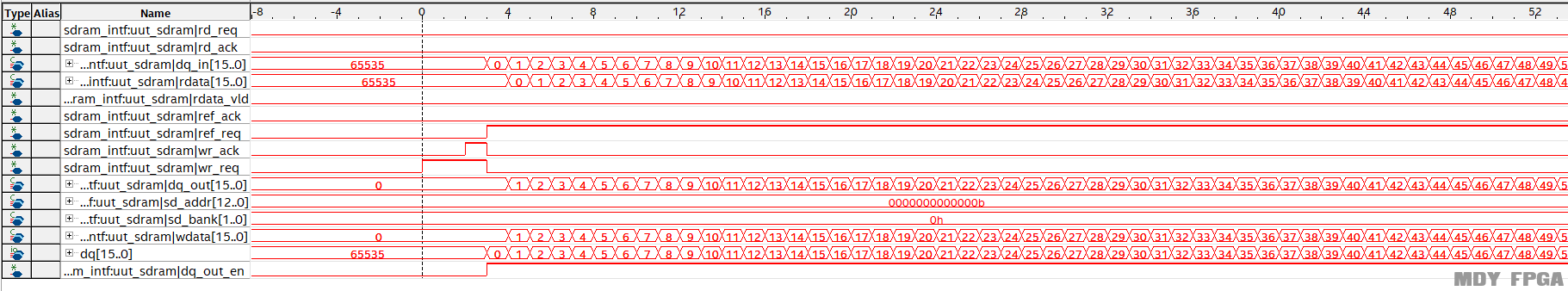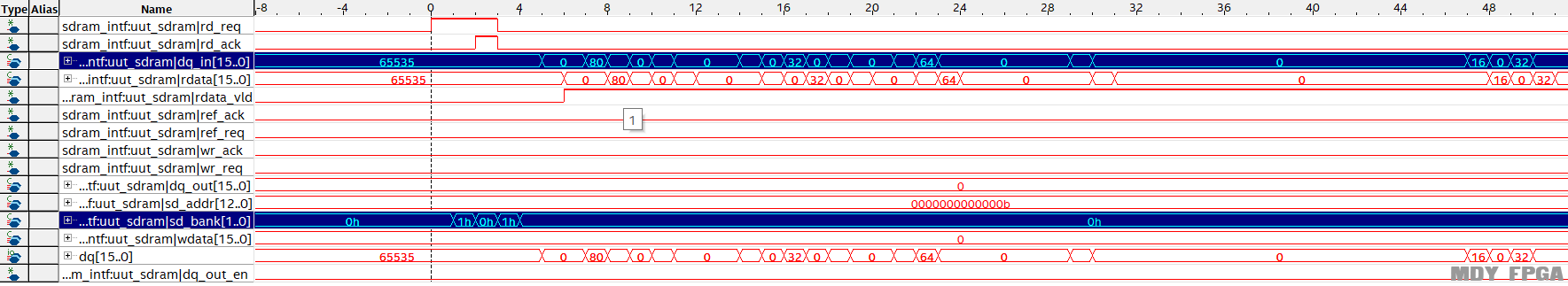拓展阅读